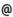## "Babes-Bolyai" University of Cluj-Napoca Faculty of Mathematics and Computer Science

 Computational algebra
 Code Semes-ter Hours: C+S+L Credits Type Section MA026 4 2+1+0 5 optional Informatică MA026 6 2+1+0 6 optional Matematici aplicate
 Teaching Staff in Charge
 Assoc.Prof. CRIVEI Septimiu, Ph.D., criveimath.ubbcluj.ro
 Lect. SACAREA Cristian, Ph.D., csacareamath.ubbcluj.ro
 Lect. SZANTO Csaba Lehel, Ph.D., szantomath.ubbcluj.ro
 Aims An introduction to Computational Algebra by a survey of applications of algebraic algorithms in Cryptography, Coding Theory and Conceptual Knowledge Processing and Representation.
 Content 1. Notions of algorithms complexity. The O notation, classes of complexity. 2. Congruences and residue classes. Euclid's algorithm, Euler's function, Chinese remainder theorem, public key cryptography: RSA, ElGamal. Electronic signature. Cryptanalysis. 3. Primality tests. Fermat, Solovay-Strassen, quadratic residues, Legendre's symbol. 4. Coding and decoding systems. Linear codes, cyclic codes, Reed-Mulles codes. Factorizing algorithms and applications in the construction of decoding systems. 5. Conceptual Knowledge Processing, Conceptual Knowledge Representation, Conceptualll browsing of data bases, applications in management and economics. Algorithms for graphical representation of knowledge.
 References 1. W. BOSMA, A. VAN DER PORTEN: Computational Algebra and Number Theory, Kluwer 1995. 2. D. BRESSOUD, S. WAGON: A Course in Computational Number Theory, Springer-Verlag 2000. 3. H. COHEN: A Course in Computational Algebraic Number Theory, Springer-Verlag 2001. 4. N. KOBLITZ : A Course in Number Theory and Cryptography, Springer-Verlag, Berlin 1994. 5. R.LIDL, G. PILZ : Applied Abstract Algebra, Springer-Verlag, Berlin1998. 6. A. M. COHEN, H. CUYPERS, H. STERK: Some Tapas of Computer Algebra, Springer-Verlag 1999 7. THE GAP GROUP: GAP - Groups, Algorithms, and Programming. Version 4.4.3. [http://www.gap-system.org] 8. T.H. CORMEN, C.E. LEISERSON, R.L. RIVEST: Introduction to Algorithms. MIT 1990. 9. D.E. KNUTH: The Art of Computer Programming, Addison Wesley Longman 1998.
 Assessment Continuous evaluation by homeworks. Project. Final exam.